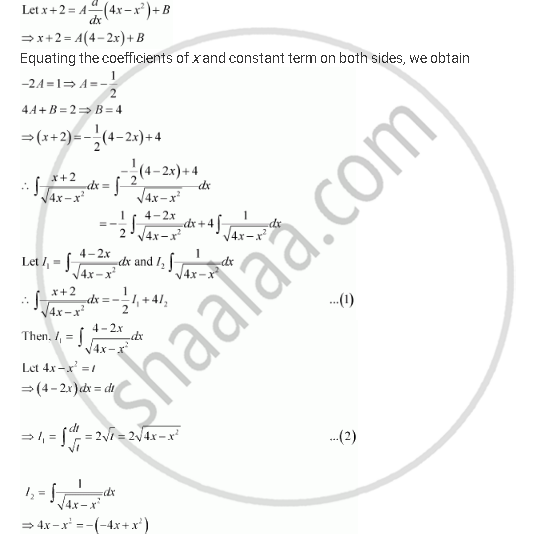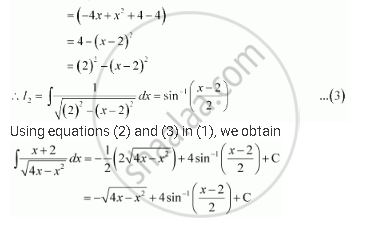Share

# Integrate the Functions (X + 2)/Sqrt(4x - X^2) - CBSE (Science) Class 12 - Mathematics

ConceptIntegrals of Some Particular Functions

#### Question

Integrate the functions (x + 2)/sqrt(4x - x^2)

#### SolutionIs there an error in this question or solution?

#### Video TutorialsVIEW ALL 

Solution Integrate the Functions (X + 2)/Sqrt(4x - X^2) Concept: Integrals of Some Particular Functions.
S